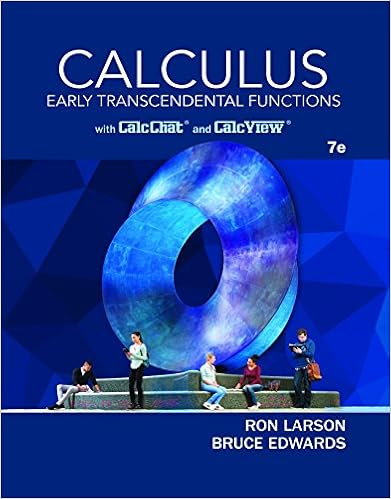x = - 5 + 3t, y = 7 - 8t, z = - 2 + 3t ; 10x + 9y...

• 3
• 100% (1) 1 out of 1 people found this document helpful

This preview shows page 1 - 2 out of 3 pages.

We have textbook solutions for you!
The document you are viewing contains questions related to this textbook.The document you are viewing contains questions related to this textbook.
Chapter 11 / Exercise 48
Calculus: Early Transcendental Functions
Edwards/LarsonExpert Verified
Group 3 Homework 2 MULTIPLE CHOICE. Choose the one alternative that best completes the statement or answers the question. Find the intersection. 1) x = - 5 + 3t, y = 7 - 8t, z = - 2 + 3t ; 10x + 9y - 9z = - 2 1) A) ( - 2, - 1, 1) B) ( - 8, 15, - C) - 82 23 , 73 23 , - 13 23 D) - 148 23 , 249 23 , - 49 5) 23
2) x = - 8 + 7t, y = 8 + 6t, z = 3 + 3t ; 4x + 7y + 10z = - 4 2) 50 6 3) = - 9t = 9 1 4) 24
Use a calculator to find the acute angle between the planes to the nearest thousandth of a radian. 5) 9x + 9y + 8z = 3 and 3x + 4y + 8z = 5 5)
Find the triple scalar product (u x v) · w of the given vectors. 6) u = 2 i - 4 j + 3 k ; v = - 10 i - 4 j + 2 k ; w = 9 i - 8 j + 10 k 6)
We have textbook solutions for you!
The document you are viewing contains questions related to this textbook.The document you are viewing contains questions related to this textbook.
Chapter 11 / Exercise 48
Calculus: Early Transcendental Functions
Edwards/LarsonExpert Verified
•••